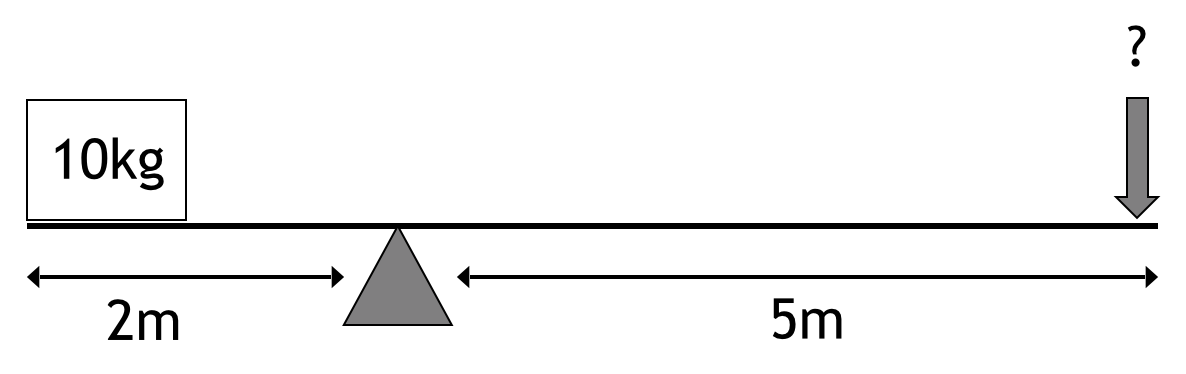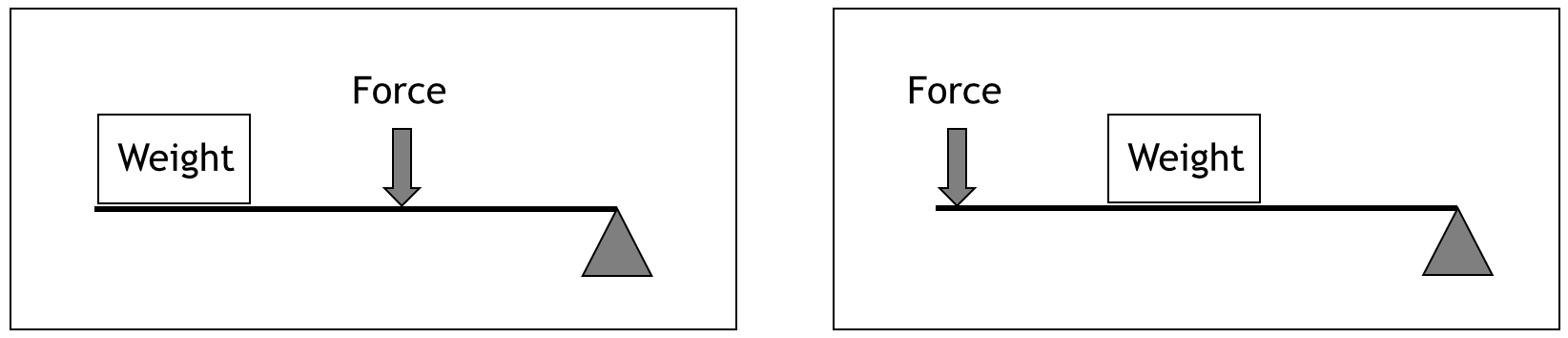Mechanical Comprehension Test

What Is A Mechanical Reasoning Test?

Mechanical reasoning tests assess your ability to apply specific mechanical or engineering principles to novel situations in order to identify the correct answer. They are usually used as part of an assessment process for engineering or technical roles.

You can think of mechanical reasoning tests as a test of applied knowledge – they measure what you know rather than your underlying potential (in the way an abstract reasoning test does). Since this is a test of applied knowledge and understanding, your performance can be improved by practice.

How Do Mechanical Reasoning Tests Work?

Mechanical reasoning tests aim to understand your ability to work with mechanical concepts. They therefore aim to challenge and stretch participants, usually through a limited time period for completing the test and/or increasingly difficult questions. Your performance will be compared to the performance of a group of similar people (known as a norm group) and your performance relative to the norm group will be used to define your mechanical reasoning ability.

Typical Mechanical Reasoning Questions

Mechanical reasoning tests assess applied mechanical ability and typically include questions referring to a range of mechanical principles. Learning and understanding these principles is essential for success on tests of mechanical reasoning.

Typically, the format of a mechanical reasoning test will include a question followed by a number of potential answers, of which only one is correct. Your job is to identify which is the correct answer. Remember, there may be cases where it is impossible to answer the question because there is insufficient data available, in which case there will be an answer option which reflects this, eg. ‘impossible to say’.

Levers

A lever is a bar that pivots at a fixed point (called the fulcrum). Levers are used to make it easier to move a load around a pivot; pushing one end of the lever down results in the upward movement of the opposite end, and this is governed by a specific equation:

w x d1 = f x d2

Where: w = weight, d1 = distance from the fulcrum to the weight, f = force needed, d2 = distance from the fulcrum to the point where the force is applied.

In the example below, the fulcrum is in the centre of the pivot, meaning that a force of 5kg will balance the weight of 5kg.Example question 1: How much force is required to lift the weight?The correct answer is a) 4kg. The calculation required is:

f = (w x d1)/d2

f = (10 x 2)/5

f = 20/5

f = 4kg

Things to watch our for in questions about levers:

• Sometimes there are questions where there is more than one weight that needs to be lifted. In this case you need to work out the force required to lift each weight separately, then add them together to get the total force required.
• Levers are usually used to reduce the amount of force needed to move an object. That said, you should not assume that this will be the case in the questions where the fulcrum may have been positioned to mean that a force greater than the weight is needed to lift it.
• Watch out for different configurations of levers like those shown below:Gears

A gear is wheel or cylinder with teeth or cogs on their outside edges. Gears are typically used in combinations of different sizes and are used in three main ways: to increase or reduce speed and force, to transmit force over a direction, or to change the direction of motion.

Gears have a number of properties that are often explored in mechanical reasoning tests:

• Gears that are connected by a chain or a belt (i.e. not physically touching) move in the same direction.
• If gears are touching (also known as meshed) they will move in opposite directions. This will alternate for each cog in a sequence; therefore for an odd number of cogs, the final gear will move in the same direction as the first, and for an even number of cogs, the final gear will move in the opposite direction to the first.
• The first gear in a sequence is known as the ‘driver.’ Subsequent gears are known as ‘followers.’ You can calculate the gear ratio by dividing the number of teeth on the follower by the number of teeth on the driver. Where there is a low gear ratio there will be more speed and less force; conversely, where there is a high gear ratio there will be less speed and more force.

Questions about gears typically use one of more of these rules and ask you to calculate the speed, direction or force of a particular gear/cog, given information about others in the sequence.

Pulleys

Pulleys typically involve a wheel with a cord/rope/belt around. They are used to raise heavy weights by changing the direction of a force applied to the rope. There are various figurations that the pulley can have, and these are important for solving the questions:

• Fixed: the load moves but the pulley does not. The force required to lift the object is equal to its weight.
• Moveable: the pulley moves with the load. The force required to lift the object is equal to half the weight.
• Combination: including more than one pulley (often, but not always, including fixed and moveable pulleys). The force is reduced but the distance increases. The force required to lift the object is equal to the weight divided by the number of ropes supporting it.

Example Question 2: How much force is required to lift the load?a) 20 kg b) 10 kg c) 5 kg d) Impossible to say.

The correct answer is c) 5 kg. The weight is 20 kg and there are 4 ropes supporting the weight. Therefore the force required to lift it is 20kg divided by 4, which is 5kg.

Take A Free Mechanical Reasoning Test

Below is a mechanical reasoning test for you to try out. It was created by WikiJob and JobTestPrep in association with psychometric experts, and is closely modelled on real tests.

The test consists of 10 questions to be answered in 10 minutes (although note there is no timer on the test itself). Our tests are slightly harder than the real thing, in order to make them sufficiently challenging practice.

You can take the test as many times as you like. Click the 'Take test' link below on either to get started.

Mechanical Reasoning Practice Test

 Questions 10 Pass Percentage 80% Time Limit 10 min

Top tips For Success In Mechanical Reasoning Tests

Practice! Since mechanical aptitude tests are tests of your applied knowledge, it is really important to ensure that you have learned and practised the types of problems likely to be included. A suggested list of topics to revise includes (but is not limited to):

• Levers
• Gears
• Pulleys
• Springs
• Screws
• Acceleration
• Gravity
• Clamps
• Shafts
• Pressure
• Friction
• Weights
• Volumes
• Conveyor belts
• Kinetic and potential energy
• Balancing scales
• Simple electrical circuits
• Applied maths
• Magnetism
• Mirrors and reflection

Take the time to understand in your own mind what problems are solved by these mechanical techniques, how they work, and how they are applied in the real world.

Learn from your practical experience. Preparing for mechanical reasoning tests does not have to be entirely theoretical. If you enjoy working with your hands, practise taking things apart to understand how they work. Fix things. Build things. Try ideas out practically and see what you learn from doing so. Find opportunities to work with tools and equipment, read technical user manuals, anything that familiarises you with the mechanical mindset and builds your knowledge.

Practising example aptitude questions is also important. Make sure that you work through some practice tests, such as those provided by Job Test Prep, to ensure you understand how to solve the questions and you can get straight on with answering them in the real test.

In your mechanical reasoning test, work quickly but carefully and double-check that you have understood the question and that your calculation is correct. Only correct answers will count towards your score, so it is worth taking the time to ensure that you have understood addressed all aspects of the question.

Further Practice

If that was useful, you may also like to try our free aptitude tests, which have been designed to simulate the questions typically used in online assessments. Although they do not contain any questions from the real tests, they are based upon them, and designed to provide practice to help you through.

If you spot any problems with our tests, please email [email protected] to let us know.

Other Articles You May Find Helpful

Improve Interview Performance